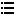fengzf2020-08-22 15:20:23

# BigDecimal先乘后除与先除后乘结果为什么差别那么大？

`discountAmount =230.00   precision=0   scale=2`
`orderAmount=730.00    precision=5   scale=2`
`BigDecimal settlementRatio = discountAmount.multiply(new BigDecimal(100)).divide(orderAmount,2,BigDecimal.ROUND_HALF_UP);`

`BigDecimal settlementRatio = discountAmount.divide(orderAmount,4,BigDecimal.ROUND_HALF_UP)`
`.multiply(new BigDecimal(100)).setScale(2,BigDecimal.ROUND_HALF_UP);`

1、首选discountAmount是计算减出来，orderAmount是从数据库取得，不能理解为什么  orderAmount的precision 跟 discountAmount的不一样？！

2、这计算结果差别也太大了吧？！

3、自己在main方法里试了一下

`System.out.println(new BigDecimal(230).setScale(2,BigDecimal.ROUND_HALF_UP).multiply(new BigDecimal(100)).divide(new BigDecimal(730).setScale(2,BigDecimal.ROUND_HALF_UP),2,BigDecimal.ROUND_HALF_UP));`

230*100/730=31.51，没毛病，Debug了一下

`230   precision=0   scale=2`
`730   precision=0   scale=2`0个评论1个牛币所有回答列表(1)扫描二维码关注最代码为好友